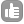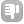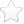1hi,when i am processing nmr data in topspin 2.1,by default we are using pk mode in ph mode.there are other modes also such as mc,ps..why we are using this one only.Thankyou asked Jul 20 '10 at 19:59sashi 413The mode ph means that a phase correction with the values of phc0 and phc1 will be applied. For 1D spectra the setting of phmode is not interpreted, only commands such as pk or fp will apply a phase correction. For multidimensional data it is. This means if phmod is set the phase correction will be execute. The modes mc and ps stand for magnitude calculation or power spectrum, this means sqrt(r^2+i^2) or (r^2+i^2) respectively. mc is used for non phase sensitive multidimensional experiments. answered Jul 23 '10 at 21:21